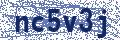Funny Status Message #113826

Funny Status Messages | Status Message Generator | Recent Comments
X has found the secret to life via this mathematical formula. To calculate, enter the following in Google: exp((-(((x-4)^2+(y-4)^2)^2))/1000)+exp((-(((x+4)^2+(y+4)^2)^2))/1000)+0.15*exp(-(((x+4)^2+(y+ 4)^2)^2))+0.15*exp(-(((x-4)^2+(y-4)^2)^2Save as Image
Related Status Messages:
• # 135616 There’s a mathematical formula for understanding women. I forgot the specifics, but at the end you divide by zero.
• # 140279 Wiki leaks: kraby patty secret formula
• # 43457 1. Go to Google Maps and click on "Get Directions." 2. Enter "USA" as your start point. 3. Enter "Japan" as your destination. 4. Go to the 31st point on your route. 5. Repost this on your status
←Rate | 10-11-2012 13:09
 Post a Comment: Comment: Name:Security Code: